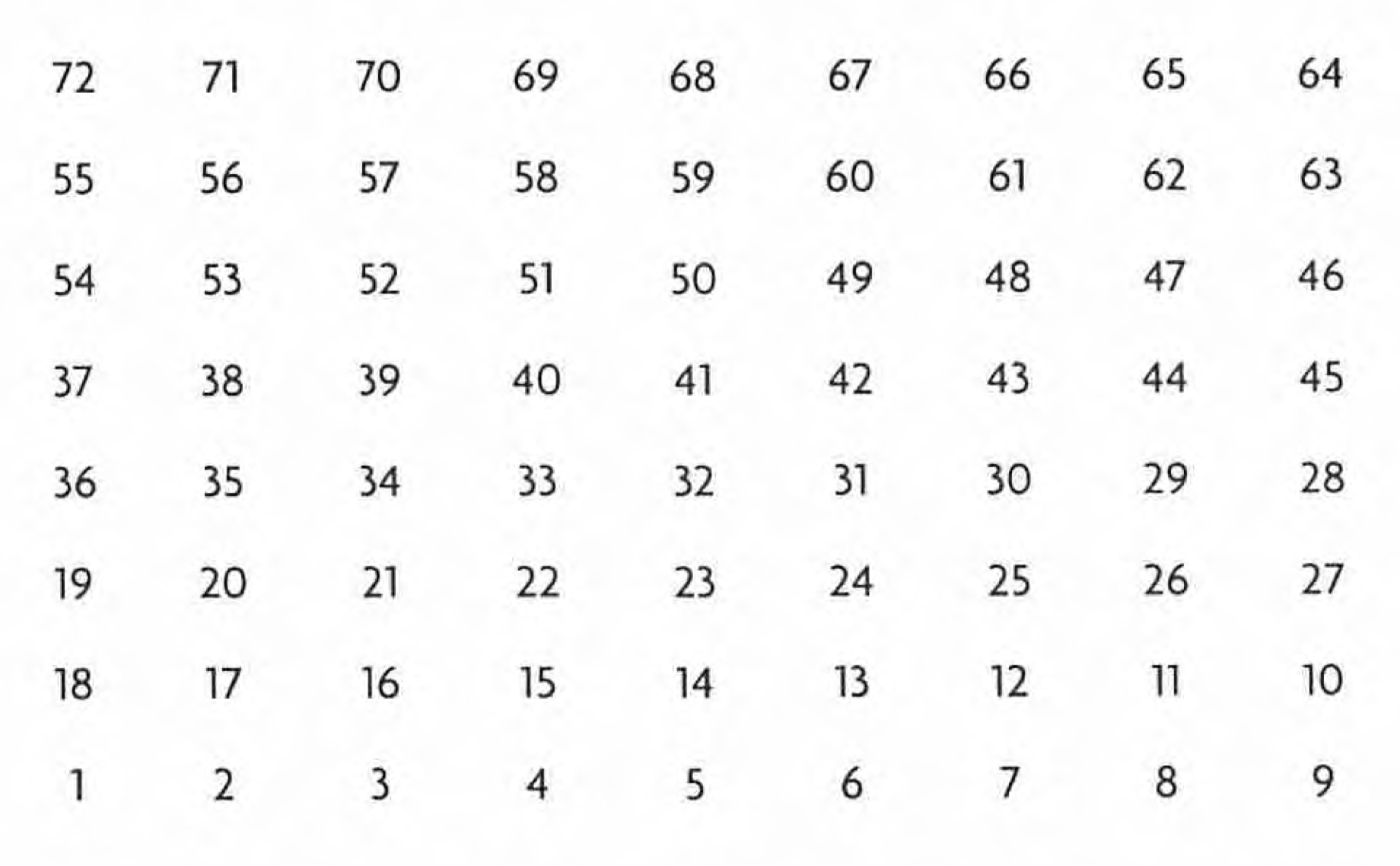## Numerology of the Game

The Indian tradition ascribes great significance to nine basic numbers, 1 to 9. Each number corresponds to a set of basic attributes, which contain keys to understanding the workings of the subtle life-force in gross manifestation. This is the teaching brought to the West by the Greek Pythagoras, a student of the Arabic version of Indian numerology.

In fact, not only do numbers count, but they give an account of the countless idea-forces working through human consciousness and the phenomenal world. They help the mind to have a definite idea of the perceptual world. In this beginningless creation there is neither first nor last, because all is one number. Actual digits are only the manifested forms, which appear as evolution starts and disappear or merge in their source with the dissolution of the phenomenal world. Everything that exists is disposed according to number, each a part (ank) of the whole (Brahman, Supreme Consciousness) manifesting himself. The whole is boundless, countless, no number, zero, shoonya — non-Being — which is the start of Leela, the cosmic play.

At the beginning is the separation of Being from non-Being. Sound, naad, is the first to evolve. Sound contains the twin aspects of sound and beat. Sound represents energy in its original vortex form. Beat is a pattern of vibration experienced in linear form. Sound creates space, and beat creates time. Each sound has a wavelength, and each wavelength exists in time. The measurement of a wavelength is the time that the wavelength takes from its origin to the end.

Plato regarded numbers as the essence of harmony and harmony as the basis of both cosmos and man. Balzac, the French novelist, called the numbers “incomprehensible agents.” According to him, distinctions between different forms of existence are differences in their qualities, quantities, dimensions, forces, attributes, and nature. These differences are not in the essence, but in the material content, which is arranged in different patterns. These patterns, when observed closely, vary only in numbers. The difference between an atom of copper and one of gold is but a difference in the number of particles they contain.The arrangement of the playing board of Leela is based on a foundation stone of numerology. The board is a numerologically balanced perfect rectangle. It contains eight horizontal rows from bottom to top. Eight is the number of the manifested universe, prakriti, which is composed of the five elements, or mahabhutas (ether, air, fire, water, and earth) and the three forces — mind, intellect, and ego (manas, buddhi, ahamkar). The vertical columns from left to right total 9, the number of the Absolute, Supreme Consciousness (the eight of prakriti plus one — Consciousness). Nine is the completion of the series of simple (basic) numbers and is therefore the number of completion. Thus there are seventy-two squares making up the field of cosmic play. Seventy-two when reduced to a simple whole number again becomes 9 (7 + 2 = 9).

In the serpentine motion of play, the start of each horizontal column begins with a number reducible to 1 and ends on a number that reduces to 9. For example, the second horizontal row begins with 10 (1 + 0 = 1) and ends on 18 (1+8 = 9). In addition, each horizontal row contains nine numbers, which when added together yield the number 9. The first row totals 45 (4 + 5 = 9), the second 126 (1 +2 + 6 = 9), the third 207 (2 + 0 + 7 = 9).

Each vertical column, except the central one, contains just two basic numbers. The first column consists of 18 (1 + 8 = 9), 19 (1 + 9 = 10 = 1), 36 (= 9), 37 (= 1), 54 (= 9), 55 (= 1), and 72 (= 9). The second vertical column yields 2s and 8s, the third 3s and 7s, the fourth 4s and 6s, and the fifth the only exception — all 5s. From here the order reverses (6s and 4s, 7s and 3s, 8s and 2s). So each vertical column, except for the middle, contains two fundamental integers, which when added together produce 1. The central column, the column of balance, contains 5s; and two 5s yield 10 or 1. In addition, the sum of each vertical column is 292, which becomes 4 (2+ 9 + 2 = 13 = 1 +3 = 4), the number of rational organization, tangible achievement, tetramorphs, the formless square.